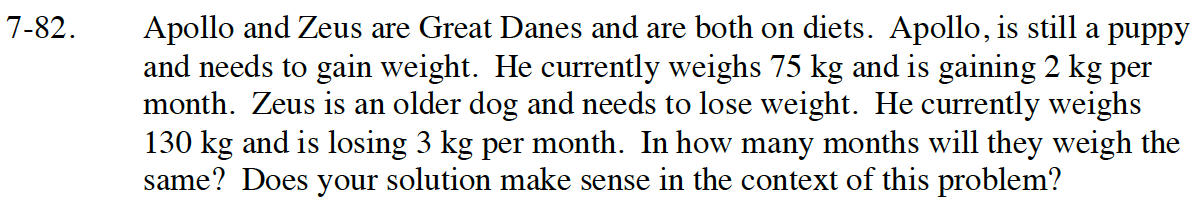7-82.

Missing problem for Book Int1 => Chapter Ch7 => Lesson 7.1.7 => Problem 7-82

Created from orphaned homework help problemUse a system of equations (2 equations), where
x = number of months and y = weight in kg.

When writing the equations use slope-intercept form
(y = mx + b). Where m is the rate of change and b is the starting weight.

Equation for Apollo is y = 2x + 75.
Equation for Zeus is y = -3x +120.

Use the equal values method to solve the system.
So 2x + 75 = -3x + 120. Solve for x.

x = 11 months